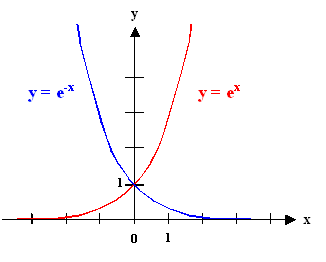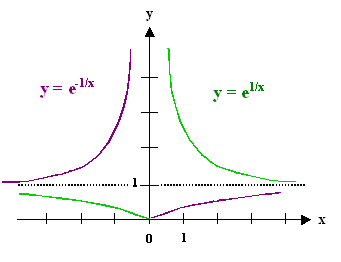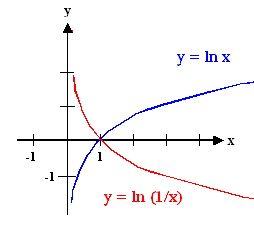Working with Exponentials and LogarithmsFirst, the graphical representation of the most important exponential curves (see math script as well)The typical curves everybody should know. The blue curve in the first quadrant (positive x values) corresponds to the energy dependence of the ubiquitous Boltzmann factor exp – (E/kT)Slightly more tricky. Note that the purple branch in the 1. quadrant corresponds to the temperature dependence of the ubiquitous Boltzmann factor exp – (E /kT)The inverted functions, e.g. y = ln x are easily pictured, too; below the y = ln x and the y = ln (1/x) functions are shown.The graphs, in case you forgot, also illustrate some basic algebraic relations, e.g.
• e– x = 1/ex
• ln x = – ln (1/x)The essential identities are
ex  =  1
e– x
(ex)y  =  ex · y

ex · ey  =  ex + y   (ex)1/y  =  ex /y

ex
ey
=  ex – y   eln x  =

ln (x · y)  =  ln x  +  ln y

ln x
y
=  ln x  –  ln y

ln xy  =  y · ln xHere some approximations.
ex  =  1  +  x
1!
+   x2
2!
+  x3
3!
+ ..

ln (1 + x)  =  x  –   x2
2
+  x3
3
–   x4
4
+ ..

ln (1 – x)  = æ è ö øWhile many equations contain exponential terms of some variable which "disappear" if you substitute the ln of the variable (as in the Arrhenius plot), we mostly prefer the lg of some observable quantity to the ln. As an example, plotting the vacancy concentration cV in an Arrhenius plot would be straight forward with the ln:On order to get a straight slope we have to switch to new variables according to
cV  = A · exp –   HF
kT

ln cV   = ln A   –   HF
kT
·  1
T

y  = ln A   –   1
T
·  x

for
y  = ln cV  x  =  1
TFor y and x we get straight line with slope HF/k and intercept = AWhat do we have to do if we want to plot lg (cV) instead of ln (cV)?We have to multiply everything with lg e = 0,4342.., because
lg x  =  (lg e) · (ln x) 1)We obtain
lg (cV)  =  0,434 · ln A  –  0,434 ·   HV
k
·   1
T1) If this puzzles you, considerWe postulate the equality
lg x  =  M · ln xand want to find a value for M. Raising everything to the power of 10 gives
10lg x  =  x  =  10M · ln xThis equation can only be fulfilled if
10M  =  ebecause then we have eln x = x as required, giving M = lg e5.3.2 Definition der Entropie und erste Anwendung5.3.4 Darstellungen der Konzentrationsfunktion5.3.3 Gleichgewichtskonzentration von atomaren Fehlstellen in KristallenLösung Übung 6.3-1Lösungen zur Übung 2.2-5

© H. Föll (MaWi 1 Skript)